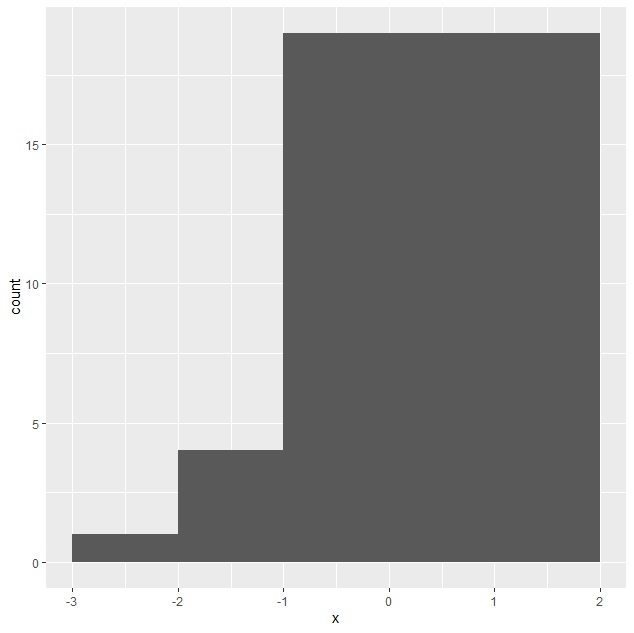# How to create histogram with varying binwidth using ggplot2 in R?

To create histogram with varying binwidth using ggplot2 in R, we can follow the below steps −

• First of all, create a data frame.

• Then, use geom_histogram function with breaks argument having varying differences.

## Example

#### Create the data frame

Let’s create a data frame as shown below −

x<-rnorm(25)
df<-data.frame(x)
df

## Output

On executing, the above script generates the below output(this output will vary on your system due to randomization) −

        x
1  -0.51481721
2  -0.15054169
3  -0.47070913
4   0.15570184
5   0.30182716
6   0.27027150
7  -1.13936013
8   1.78922492
9  -1.80448026
10 -0.50952246
11  2.06501828
12  0.18205980
13 -1.09307457
14 -0.69088644
15 -0.01556974
16 -0.18344297
17  0.56017703
18  0.90763553
19  1.63306307
20  0.83391193
21  0.09490660
22 -1.42484122
23 -0.52230854
24  0.77694351
25 -2.17216831

Create histogram with varying binwidth using ggplot2

Using geom_histogram function with breaks argument having varying differences to createthe histogram having different binwidths as shown below −

x<-rnorm(25)
df<-data.frame(x)
library(ggplot2)
ggplot(df,aes(x))+geom_histogram(breaks=c(-3,-2,-1,2))

## OutputUpdated on: 15-Nov-2021

428 Views